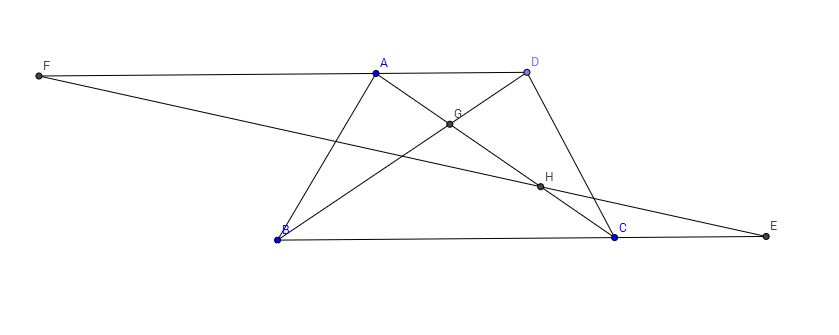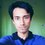# Trapezoid=Parallelogram! What?I simply love mathematical fallacies. They are a great source of mathematical entertainment, they're fun to think about and I love the looks on my friends' faces when they are stuck thinking about a mathematical fallacy.

On this post we're going to 'prove' that all trapezoids are actually parallelograms.

So, what is a trapezoid? A trapezoid is a quadrilateral [a polygon with exactly $4$ sides] with at least one pair of parallel sides. And a parallelogram is a quadrilateral with two pairs of parallel sides. Notice that according to this definition all parallelograms are trapezoids but all trapezoids are not parallelograms. Even though we know this, we're going to 'prove' otherwise. You know, just for fun!

As a quick aside you should know that what we've just called a trapezoid is called a trapezium outside of North America. This is really confusing because a in North America, a trapezium means something else [a quadrilateral with no parallel sides]. We don't want to get lost in a sea of definitions. So we're going to stick to the trapezoid-definition from the previous paragraph.

Let's begin!Alt text

Let's call $ABCD$ a trapezoid with $AD || BC$. $BC$ is extended to $E$ such that $CE=AD$. Similarly $DA$ is extended to $F$ such that $AF=BC$.

I'm feeling a little lazy. So, I'm going to write $FA=BC=b$ and $AD=CE=a$. Also, $x=AG$, $y=GH$ and $z=CH$.

By now you should start to notice that there are some pairs of similar triangles here.

$\triangle CBG$ and $\triangle ADG$ are similar.

So, $\frac{BC}{AD}=\frac{CG}{AG}$

$\Rightarrow \frac{b}{a}=\frac{y+z}{x}$

So, $x=\frac{a(y+z)}{b} \cdots (1)$

Triangles $AFH$ and $CEH$ are similar. That means,

$\frac{AF}{CE}=\frac{AH}{CH}$

$\frac{b}{a}=\frac{x+y}{z}$.

So, $z=\frac{a(x+y)}{b} \cdots (2)$

Now we're going to subtract $(1)$ from $(2)$.

We're left with:

$z-x=\frac{a(x-z)}{b}$

$\Rightarrow |(z-x)|=|\frac{a(x-z)}{b}|$

$\Rightarrow |(z-x)|=|\frac{a}{b}|\times |(z-x)|$

$\Rightarrow |\frac{a}{b}|=1$

$\Rightarrow|a|=|b|$.

In other words, $AD=BC$. You know what this means, right? This means $ABCD$ is in fact a parallelogram. And that means all trapezoids are parallelograms.

So, what went wrong? I advise you stop reading now and think about this for a while. The solution is very simple and it's literally right under your noses [is this a correct usage of the word 'literally'?]. I also warn you that if you choose to read on you might feel like, "Darn! That was easy! I could have figured it out ."

Don't say you haven't been warned!

Congratulations to those who have figured it out or at least thought about it for a while. For those who were reading on, read on!

So, what is the fishiest step in the entire proof? The absolute value operator sure came out of nowhere! It just appeared with no rhyme or reason. When you see something fishy, that something fishy has a purpose. Here, the absolute value operator is disguising something. Let's do the steps without taking the absolute value operator. What do we get? We get this:

$\frac{a}{b}=-1$

Okay, this is weird. Because $a$ and $b$ are the lengths of the bases of the trapezoid and by convention, they're positive. So their ratio has to be positive. We have this negative ratio because we divided both of the sides by $(z-x)$. When do we see weird stuff happening after a division? When the divisor is $0$! [this not zero factorial, I'm just excited!]

$(z-x)$ is indeed $0$ and that's why when we divided the equation by it, we were left with a negative ratio that was always supposed to be positive.

Division by zero certainly doesn't destroy the space-time continuum, but it does turn all trapezoids into parallelograms!

So, $(z-x)$ is really zero? You're going to take my word for it? Right after I just presented a fallacious proof? Prove to yourself that $(z-x)$ really does equal zero or in other words, $z=x$. Post your proof in the comments section if you feel like it.

Source: I read this on Cut The Knot. This is a really great site for geometry.Note by Mursalin Habib
7 years, 4 months ago

This discussion board is a place to discuss our Daily Challenges and the math and science related to those challenges. Explanations are more than just a solution — they should explain the steps and thinking strategies that you used to obtain the solution. Comments should further the discussion of math and science.

When posting on Brilliant:

• Use the emojis to react to an explanation, whether you're congratulating a job well done , or just really confused .
• Ask specific questions about the challenge or the steps in somebody's explanation. Well-posed questions can add a lot to the discussion, but posting "I don't understand!" doesn't help anyone.
• Try to contribute something new to the discussion, whether it is an extension, generalization or other idea related to the challenge.
• Stay on topic — we're all here to learn more about math and science, not to hear about your favorite get-rich-quick scheme or current world events.

MarkdownAppears as
*italics* or _italics_ italics
**bold** or __bold__ bold
- bulleted- list
• bulleted
• list
1. numbered2. list
1. numbered
2. list
Note: you must add a full line of space before and after lists for them to show up correctly
paragraph 1paragraph 2

paragraph 1

paragraph 2

[example link](https://brilliant.org)example link
> This is a quote
This is a quote
    # I indented these lines
# 4 spaces, and now they show
# up as a code block.

print "hello world"
# I indented these lines
# 4 spaces, and now they show
# up as a code block.

print "hello world"
MathAppears as
Remember to wrap math in $$ ... $$ or $ ... $ to ensure proper formatting.
2 \times 3 $2 \times 3$
2^{34} $2^{34}$
a_{i-1} $a_{i-1}$
\frac{2}{3} $\frac{2}{3}$
\sqrt{2} $\sqrt{2}$
\sum_{i=1}^3 $\sum_{i=1}^3$
\sin \theta $\sin \theta$
\boxed{123} $\boxed{123}$

## Comments

Sort by:

Top Newest

The fact that $x = z$ is right in your "proof"! When we find that $z = \frac{a(x + y)}{b}$ and $x = \frac{a(z + y)}{b}$, we can rearrange to get that $bz - ax = ay = bx - az \to (a + b)x = (a + b)z \to x = z$.

I enjoy fallacies, too, and this was a nice one I'd never seen before. Thanks for the post :)

- 7 years, 4 months ago

Log in to reply

Actually...My friend had already shown the same thing to me and challenged me to point out the fault.....spent 5 minutes and got z=x...just as you did!!!

- 7 years, 1 month ago

Log in to reply

Here's an example of the trapezoid-trapezium confusion. I wanted to add the #Trapezoid tag, but Brilliant tags have a #Trapezoid(nosidesparallel) as default. You see what I mean?

- 7 years, 4 months ago

Log in to reply

There's a debate about the definitions of Trapezium and Trapezoid, mainly due to a swap in the definitions in Hutton's Mathematical Dictionary in 1795 for the United States. We have decided to go with trapezium = 1 side parallel, and also include this in the details and assumptions (and also the tag name), to try and reduce confusion. I have added the tag #Trapezium(1sideparallel).

As always, it's best to know the definitions of what the terms are, when you are communicating with others. Another common mis-definition is that the Dutch consider 0 to be positive (oh the horror!), and Euclid considers a rhombus to not include rectangles (though I do), and some kindergarden teachers which say that "A square tilted on it's side is a diamond, not a square" (and thereby destroying the idea of congruent figures of these kids).

Staff - 7 years, 4 months ago

Log in to reply

It was a really nice post...thanks for sharing it!!

- 7 years, 1 month ago

Log in to reply

And thank you for reading!

- 7 years, 1 month ago

Log in to reply

Nice job, again! Great article, very well-explained.

- 7 years, 4 months ago

Log in to reply

Thanks!

- 7 years, 4 months ago

Log in to reply

When I read this quickly my thought was that you defined $FA=BC=x$ and $AG=x$, so you misused variables. Just pointing out that there is a typo there.

Nice article, I especially like your commentary :)

- 7 years, 4 months ago

Log in to reply

Thanks for pointing that out! Now it's fixed. I meant $FA=BC=b$. I should have proofread it.

- 7 years, 4 months ago

Log in to reply

Really an appreciable one $+$ Good sense of humour. Another typo :$8$th line$---->$ "but all parallelograms are not trapezoids".

- 7 years, 3 months ago

Log in to reply

Darn it! I'm going to try and reduce the number of typos in my next posts. Thanks for pointing it out!

- 7 years, 3 months ago

Log in to reply

Good one. I enjoyed it !

- 7 years, 4 months ago

Log in to reply

Very Nice

- 7 years, 3 months ago

Log in to reply

nice article. But side ratios of corresponding sides taken here is not correct. proportionality exist only among CORRESPONDING SIDES. Here Corresponding side of CG is DG not AG. As for similarity corresponding angles should be same.

- 7 years, 3 months ago

Log in to reply

Nope! $\angle GBC= \angle GDA$. That means corresponding sides are $CG$ and $AG$ respectively.

- 7 years, 3 months ago

Log in to reply

let see, we have two similar triangles,GBC & GDA (draw accordingly in anti cloclwise manner). The interior angles B&D , C&A, and G&G are CORRESPONDING angles. then notice that is CG & DG are CORRESPONDING SIDES?. if yes the yes, then please make me correct about the rules of corresponding sides.

- 7 years, 3 months ago

Log in to reply

I'm not sure what you're trying to say by

The interior angles B&D , C&A, and G&G are CORRESPONDING angles. then notice that is CG & DG are CORRESPONDING SIDES?.

Let me help you here. $AD$ and $BC$ are two parallel lines and $BD$ is the transversal. $\angle GBC$ and $\angle GDA$ are alternate interior angles. So they are equal. Are you following me so far?

Now when we say that two triangles are similar, we say that the ratios of the corresponding sides are equal. One way to find the corresponding sides is: taking the sides that are opposite to the equal angles.

The side opposite to $\angle GBC$ is $CG$. And the side opposite $\angle GDA$ is $AG$. Since $\angle GBC=\angle GDA$, $CG$ and $AG$ are corresponding sides.

I hope this helps. And let me know if you have any more questions.

- 7 years, 3 months ago

Log in to reply

This article is amazing :) Really well done Mursalin!

- 7 years, 3 months ago

Log in to reply

really i enjoyed it

- 7 years, 3 months ago

Log in to reply

Mursalin, can you email me on snehalshekatkar@gmail.com?

- 7 years, 3 months ago

Log in to reply

×

Problem Loading...

Note Loading...

Set Loading...